# Math in Focus Grade 4 Chapter 10 Practice 3 Answer Key Horizontal and Vertical Lines

This handy Math in Focus Grade 4 Workbook Answer Key Chapter 10 Practice 3 Horizontal and Vertical Lines detailed solutions for the textbook questions.

## Math in Focus Grade 4 Chapter 10 Practice 3 Answer Key Horizontal and Vertical Lines

Question 1.
$$\overline{A B}$$ is perpendicular to $$\overline{B C}$$.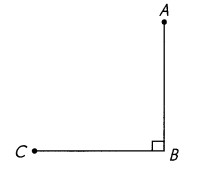If $$\overline{A B}$$ is a vertical line segment, what do you know about $$\overline{B C}$$?
AB is a vertical line segment and BC is horizontal line segment.

Question 2.
a. $$\overline{D E}$$ is a vertical line segment. Draw a horizontal line segment through point D and label it $$\overline{D F}$$.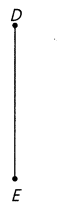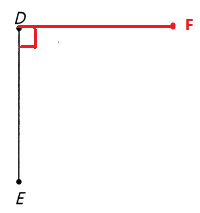Drawn a horizontal line segment through point D and label it as DF.

b. What do you know about the angle formed by $$\overline{D E}$$ and $$\overline{D F}$$?
The angle formed by DE and DF is 90°.

Complete.

Question 3.
a. $$\overline{M N}$$ is a horizontal line segment. Draw a vertical line segment through point O to meet $$\overline{M N}$$ and label the point P.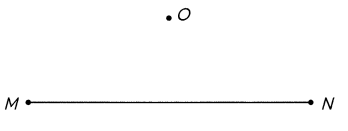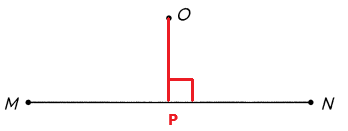b. What do you know about $$\overline{M N}$$ and $$\overline{O P}$$?
MN is a horizontal line segment and OP is a vertical line segment.

c. How many right angles are formed by $$\overline{M N}$$ and $$\overline{O P}$$?
Two right angles are formed by MN and OP.

Question 4.
a. $$\overline{P Q}$$ is a horizontal line segment.
Draw a vertical line segment at point P.
Name it $$\overline{P R}$$. Then draw a vertical line segment at point Q.
Name it $$\overline{Q S}$$.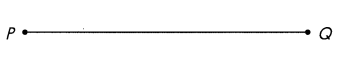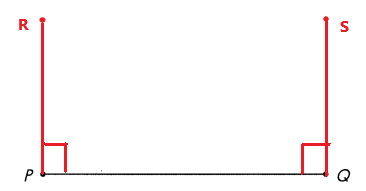Drawn a vertical line segment at point P and named it as PR.
Drawn a vertical line segment at point Q and named it as QS.

b. What do you know about $$\overline{P R}$$ and $$\overline{Q S}$$? Check with a drawing triangle and a straightedge.
Both PR and QS are parallel to each other.

Complete.

Question 5.
a. $$\overline{A B}$$ is a horizontal line segment and $$\overline{C D}$$ is a vertical line segment. At point D, draw a line segment parallel to $$\overline{A B}$$. Name it $$\overline{D E}$$.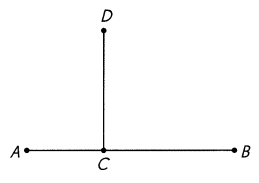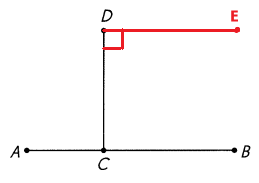At point D, drawn a line segment parallel to AB and named it as DE.

b. What do you know about $$\overline{C D}$$ and $$\overline{D E}$$?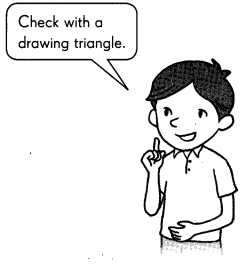Both CD and DE are perpendicular to each other.

Complete.

Question 6.
ABCD is a whiteboard fixed to the wall.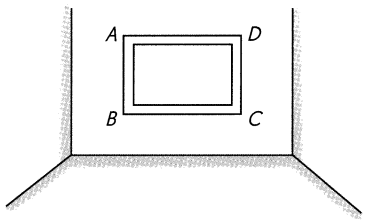Name the vertical and horizontal line segments on the whiteboard.
Vertical line segments: ____
Horizontal line segments: ____